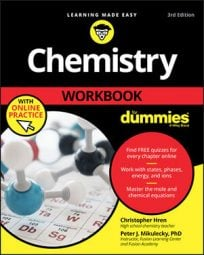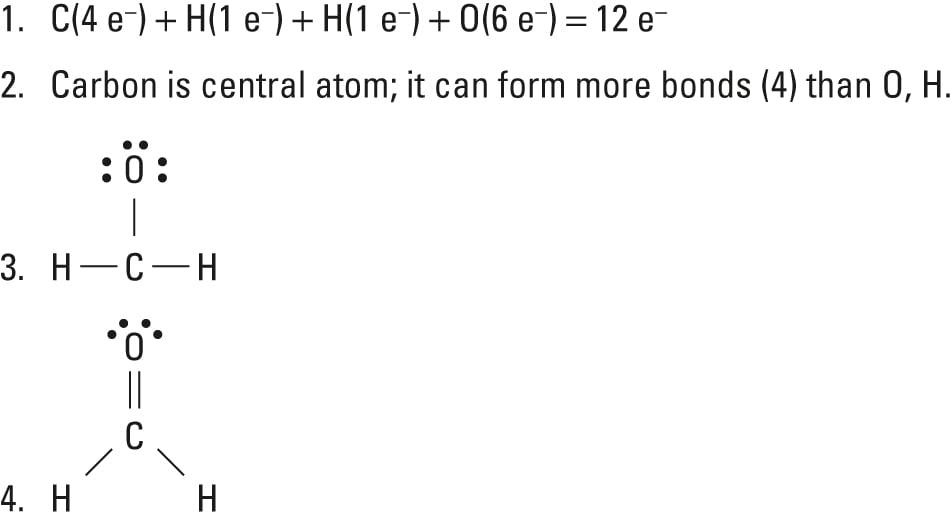##### Chemistry Workbook For Dummies with Online PracticeGetting through a chemistry class involves a range of science skills and procedures. You use exponential and scientific notation, analyze atomic structures, name compounds, convert to and from moles, and draw Lewis dot structures. Is there anything you don't do in chemistry?

## Basic exponential and scientific notation used in chemistry

In chemistry, you often use exponential notation, which is simply using exponents to express a number. Scientific notation is a specific form of exponential notation that uses a power of 10 and a number between 1 and 10. Here’s the format, where A is between 1 and 10 and B is an integer:

A × 10B

When you do calculations with measurements, you must make sure that your answer suggests no greater precision than was present in the original measurements. Here are some guidelines for writing your answers in scientific notation:

• For addition and subtraction: Use exponential notation to express both numbers using the same power of 10, and then do the math. Round the sum or difference to the same number of decimal places as held by the measurement with the fewest decimal places. If your answer isn’t in scientific notation (the first number in your answer isn’t between 1 and 10), convert as needed.

• For multiplication and division: Express both numbers in scientific notation so you can clearly see how many significant figures each number has. Round the product or quotient to the same number of significant figures as held by the measurement with the fewest significant figures.

## Analyzing atomic structure for chemistry

Chemistry deals with atoms, which are composed of subatomic particles that differ in mass and charge. Altering the numbers of these particles can alter the element or the isotope identity of an atom. Here are some subatomic facts:

• Subatomic particles:

• Proton, p+: 1 atomic mass unit (amu), +1 charge

• Electron, e: 1/1,836 amu, –1 charge

• Neutron, n0: 1 amu, 0 charge

• Atomic number:

• Equals the number of protons

• Determines the element

• Mass number:

• Equals the number of protons plus neutrons

• Determines the isotope

## How to name a chemical compound

Someone who doesn’t know chemistry may think that compounds should already have names, but you know differently. The following steps take you through the process of writing the name of any chemical you’re likely to encounter in a general chemistry class:

1. Does the formula begin with an H?

If so, the compound is most likely an acid. Use the rules for naming an acid.

2. Does the formula contain a metal (not hydrogen)?

If it doesn’t, you need to use prefixes when naming because you’re dealing with a molecular (covalent) compound. Be sure to change the ending of the second element to -ide. If there is a metal, you’re dealing with an ionic compound — proceed to Step 3.

3. Is the cation a transition metal (Group B) or a metal with a variable charge?

If the cation is a Group B metal (or other metal of variable charge, like tin), you need to use a Roman numeral to specify its charge. If the cation isn’t a transition metal and you know the charge, you don’t need to specify the charge.

4. Is the anion a polyatomic ion?

If so, you write the name of the polyatomic ion. If the anion isn’t a polyatomic ion, you use an ide ending.

## Mole conversion tips for chemistry

In a general chemistry class, you usually end up having to perform a lot of conversions involving moles (mol). Whether you’re converting from moles to grams, moles to volume, or moles to particles (atoms or molecules), use this quick guide to remind you of how to do each type of mole conversion:

• Converting from mass (grams) to moles: Divide your initial mass by the molar mass of the compound as determined by the periodic table.

• Converting from moles to mass (grams): Multiply your initial mole value by the molar mass of the compound as determined by the periodic table.

• Converting from volume (liters) to moles: Divide your initial volume by the molar volume constant, 22.4 L.

• Converting from moles to volume (liters): Multiply your mole value by the molar volume constant, 22.4L.

• Converting from particles (atoms, molecules, or formula units) to moles: Divide your particle value by Avogadro’s number, 6.02×1023. Remember to use parentheses on your calculator!

• Converting from moles to particles (atoms, molecules, or formula units): Multiply your mole value by Avogadro’s number, 6.02×1023.

• Mole-to-mole conversions: Use the coefficients from your balanced equation to determine your conversion factor. Be sure your units cancel out so you end up with the correct mole value.

## Drawing Lewis dot structures for chemistry

In chemistry, drawing Lewis dot structures can be challenging, but they provide a wealth of information about the molecules they represent. Remember that Lewis dot structures are drawn for covalent (molecular) compounds that share electrons. Follow these simple steps to correctly draw a Lewis dot structure:

1. Add up the total number of valence electrons found in the entire compound.

Don’t forget to include any positive or negative charges when determining this.

2. Draw the simple structure (skeleton structure) of the compound by connecting everything with single bonds only.

3. Add electrons to all the noncentral atoms.

Note that most atoms want eight total electrons, so atoms that have only one bond will each need to receive six electrons. However, hydrogen wants only two electrons; each bond counts as two shared electrons, so don’t add any electrons to hydrogen.

4. Put any unused electrons on the central atom.

If all atoms (except hydrogen) now have eight electrons, you’re done.

5. If one or more atoms do not have eight electrons, you must form double or triple bonds between them.

Keep in mind that each bond counts for two shared electrons.

6. If all atoms now have eight electrons around them, you’re done. If you have valence electrons left over, add them to the central atom, even if it violates the octet rule.